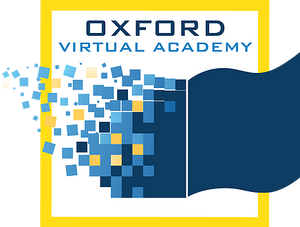Math 3 Math-U-See (Hybrid)

Math-U-See: Once students have mastered the concepts of addition and subtraction (covered in Alpha and Beta), they are ready for multiplication. Gamma teaches single-digit facts and multiple-digit multiplication skills. Major Concepts and Skills Include: Using strategies based on place value and properties of operations to multiply, Fluently multiplying any combination of whole numbers, Solving for an unknown factor, Solving abstract and real-world problems involving addition, subtraction, and multiplication, Measuring and computing area, Relating concepts of area to addition and multiplication, Additional Concepts and Skills: Skip counting as a precursor to multiplication, Adding and subtracting time in hours and minutes, Multiplying, adding, and subtracting U.S. currency and standard units of measure, Representing, recording, and interpreting data, Understanding of basic fractions, and estimating and solving measurement problems.

* Prerequisites: None

* This course includes an optional learning experience.